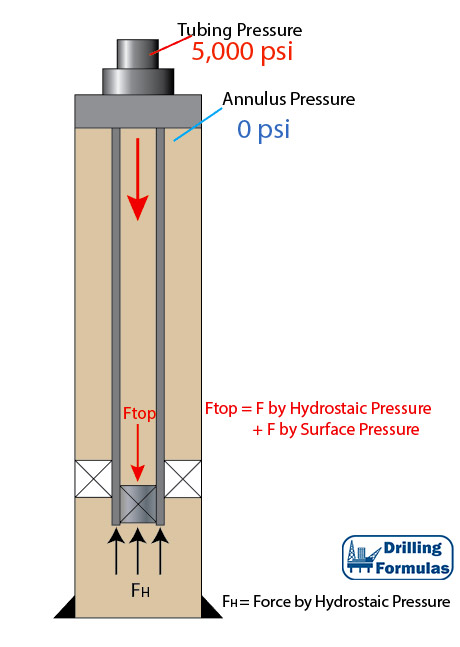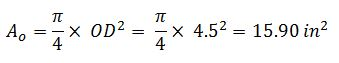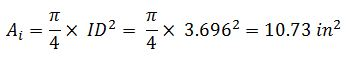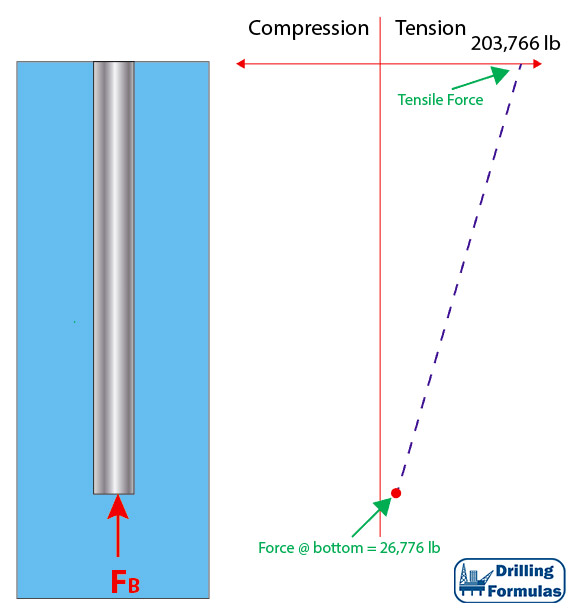# Piston Force on Closed-Ended Tubular (Plugged Tubular)

According to the previous post (piston force on open-ended tubular), applied surface pressure will reduce tensile force on surface. In this article, this is an analysis on the piston force on a plugged tubular string and the details are shown below;

Tubing Detail

• 5” Tubing
• ID of tubing = 3.696”
• Packer seal bore OD = 5.25”
• Weight per length = 17.7 lb/ft
• Total Length = 10,000 ft
• Plugged tubing depth = 10,000 ft
• Fluid density = 10 ppg
• Tubing is free to move in the packer
• Applied surface pressure = 5,000 psi

Figure 1 shows the wellbore schematic. Applied pressure (5,000 psi) will cause a piston effect to push the tubing. Therefore, at the bottom of tubing buoyancy and piston force will act in an upwards direction (compression).Figure 1 – Wellbore Schematic

Pressure at the bottom

Since there is a plug which will stop surface pressure going down to the bottom of tubing, the force acting up (compression force) is only hydrostatic pressure acting against the cross sectional area.

Pressure at the bottom (psi) = Hydrostatic Pressure (psi)

Pressure at the bottom (psi) = 0.052×10×10,000 = 5,200 psi

Force at the bottom

Force at the bottom (lb) = Pressure at the bottom (psi) × cross sectional area of pipe (in2)Force at the bottom (lb) = 5,200 psi × 15.9 = 82,680 lb (compression ⇑(+))

Total area is used for this calculation because the pressure pushes upwards against plug and cross section area of pipe.

Pressure at top of plug

Pressure on top of plug is equal to summation of applied surface pressure and hydrostatic pressure.

Pressure at top of plug (psi) = Hydrostatic Pressure (psi) + Applied Surface Pressure (psi)

Pressure at top of plug (psi) = (0.052×10×10,000) + 5,000 = 10,200 psi

Force at the top of plug

Force at the top of plug (lb) = Pressure at the top of plug (psi) × internal cross sectional of pipe (in2)Force at the top of plug (lb) = 10,200 × 10.73 = 109,446 lb (tensile force ⇓ (-))

Total Force acting at the bottom

Total Force acting at the bottom = Force acting on top of plug + Force acting at the bottom of plug

Total Force acting at the bottom (lb) = (-109,446 lb) + (82,680 lb) = -26,776 lb (tensile force ⇓ (-))

It is a tensile force due to applied surface pressure on the plugged string.

Total Force on the surface

Force at surface is a summation of tensile force caused by tubing weight and tensile force from the applied surface pressure

Force at surface (lb) = (- 17.7 × 10,000) + (-26,776) = -203,766 lb (tensile force ⇓ (-))

Figure 2 illustrates force distribution based on the given information.  It is seen that none of tubular section is exposed to compression force.Figure 2 – Force Distribution Diagram

Note: for this calculation, the assigned signs and directions of force and pressure are as follows;

Compression force = ⇑(+)

Tensile force = ⇓(-)

Shorten in Length = (-)

Elongate in length = (+)

The signs are based on the Lubinski’s study.

Lubinski, A., & Althouse, W. S. (1962, June 1). Helical Buckling of Tubing Sealed in Packers. Society of Petroleum Engineers. doi:10.2118/178-PA

References

Jonathan Bellarby, 2009. Well Completion Design, Volume 56 (Developments in Petroleum Science). 1 Edition. Elsevier Science.

Wan Renpu, 2011. Advanced Well Completion Engineering, Third Edition. 3 Edition. Gulf Professional Publishing.

Ted G. Byrom, 2014. Casing and Liners for Drilling and Completion, Second Edition: Design and Application (Gulf Drilling Guides). 2 Edition. Gulf Professional Publishing.

Lubinski, A., & Althouse, W. S. (1962, June 1). Helical Buckling of Tubing Sealed in Packers. Society of Petroleum Engineers. doi:10.2118/178-PA

Share the joy
Tagged , , , , , , , , . Bookmark the permalink.